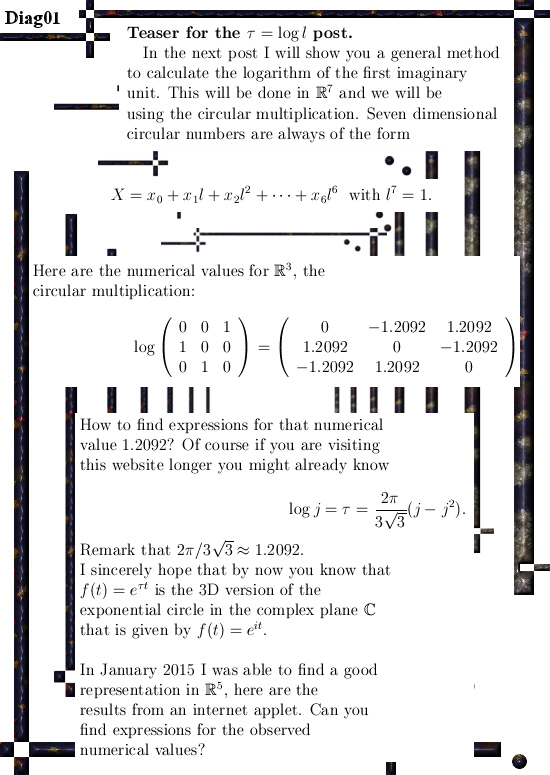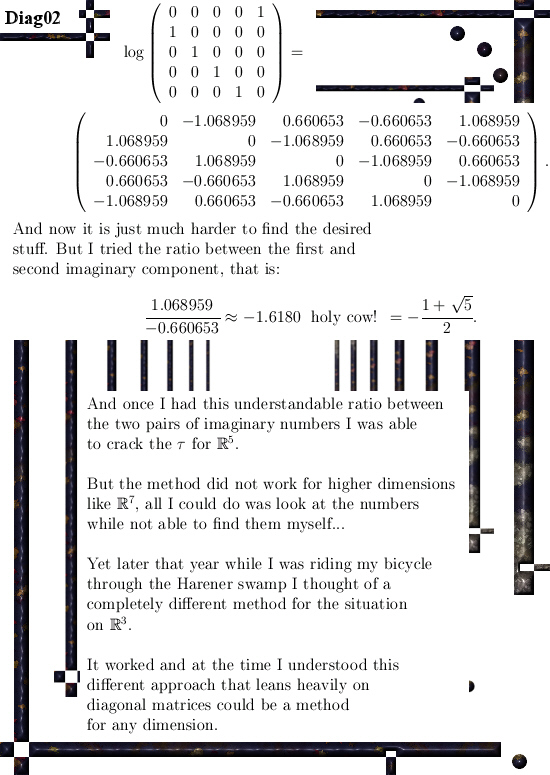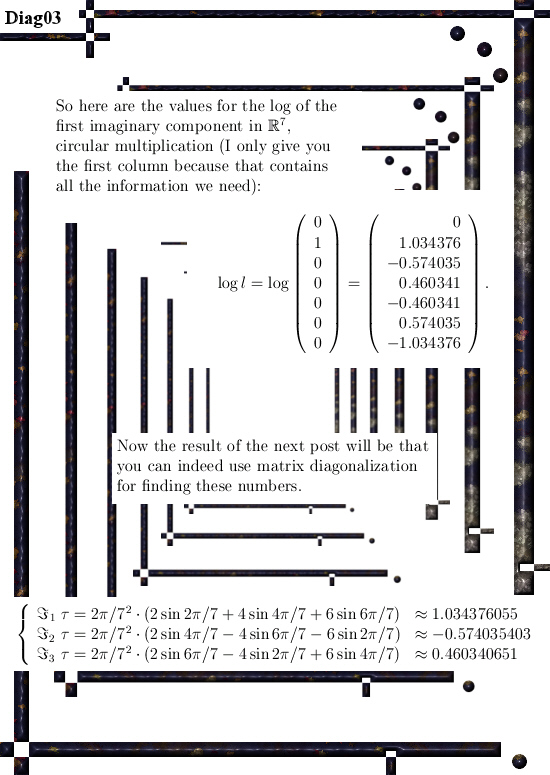# Intro to the calculation of the seven dimensional number tau (circular version).

All details will be in the next post but I succeeded into using matrix diagonalization in order to find this seven dimensional number tau.
For people who do not understand what a number tau is, this is always the logarithm of an imaginary unit. Think for example at the complex plane and her imaginary unit i. The number tau for the complex plane is log i = i pi/2.

The problem with finding numbers tau becomes increasingly difficult as the number of dimensions rise. I remember back in the year 2015 just staring at all those matrices popping up using internet applets like the next one:

Matrix logarithm calculator (it uses the de Pade approximation)
http://calculator.vhex.net/calculator/linear-algebra/matrix-logarithm

Yet back in the year 2015 I was riding on my noble iron horse (a cheap bicycle) through the swamps surrounding the village of Haren and suddenly I had a good idea. Coming home I tried the idea of matrix diagonalization out in 3 dimensions and it worked.

I even wrote a post about it on 23 Nov 2015:

Integral calculus done with matrix diagonalization.

Now I think that most readers who visit this website are familiar with the concept of finding a diagonal matrix D containing all eigenvalues of a given matrix M. Once you have the eigenvalues you can calculate the eigenvectors and as such craft your matrix C containing all eigenvectors.
You can write the stuff as next: D = C^-1 M C.

Suppose you don’t know what M is but I give you the matrices D, C and the inverse of C. Can you find the matrix M?

Yes that is a beerwalk, all you have to do is calculate M = C D C^-1 and you are good to go.

But with the logarithm comes a whole lot of subtle things for making the right choice for the eigenvalues that you place inside the diagonal matrix D. It turns out you only get the desired result if you use arguments in the complex plane between minus and plus pi.
This is caused by the fact that you always need to make a cut in the complex plane if you want to work with the complex logarithm; but it is a bit surprising that only the cut where you leave out all real negative numbers (and zero of course) makes the calculation go perfect and in all other cases it ends in utter and total disaster.

In the next three pictures I show you some screen shots with numerical values of matrix representations and the logarithm of those matrix representations.

The goal is to find mathematical expressions for the observed numerical values that are calculated via the above mentioned de Pade approximation. We don’t want only numerical approximations but also catch the stuff in a mathematical formulation.

At the end of the third picture you see the end result.So it took some time to find this result, I wasted an entire week using the wrong cut in the complex plane. And that was stupid because I had forgotten my own idea when riding my noble iron horse through the Harener swamps…

The result for the seven dimensional number tau (circular version) as calculated in the next post is a blue print for any dimension although I will never write stuff down like in a general dimension setting because that is so boring to read.

Ok, see you around my dear reader.